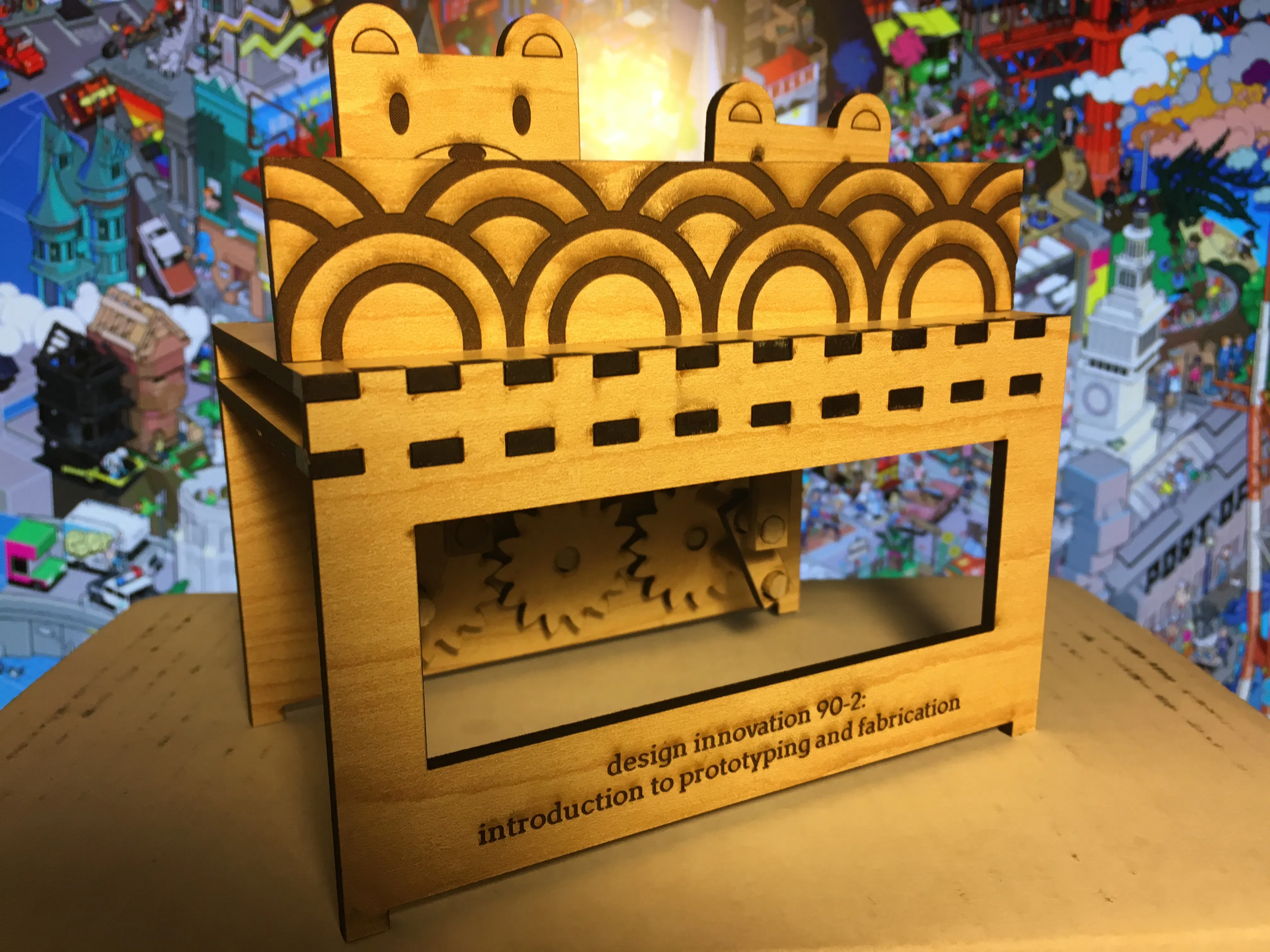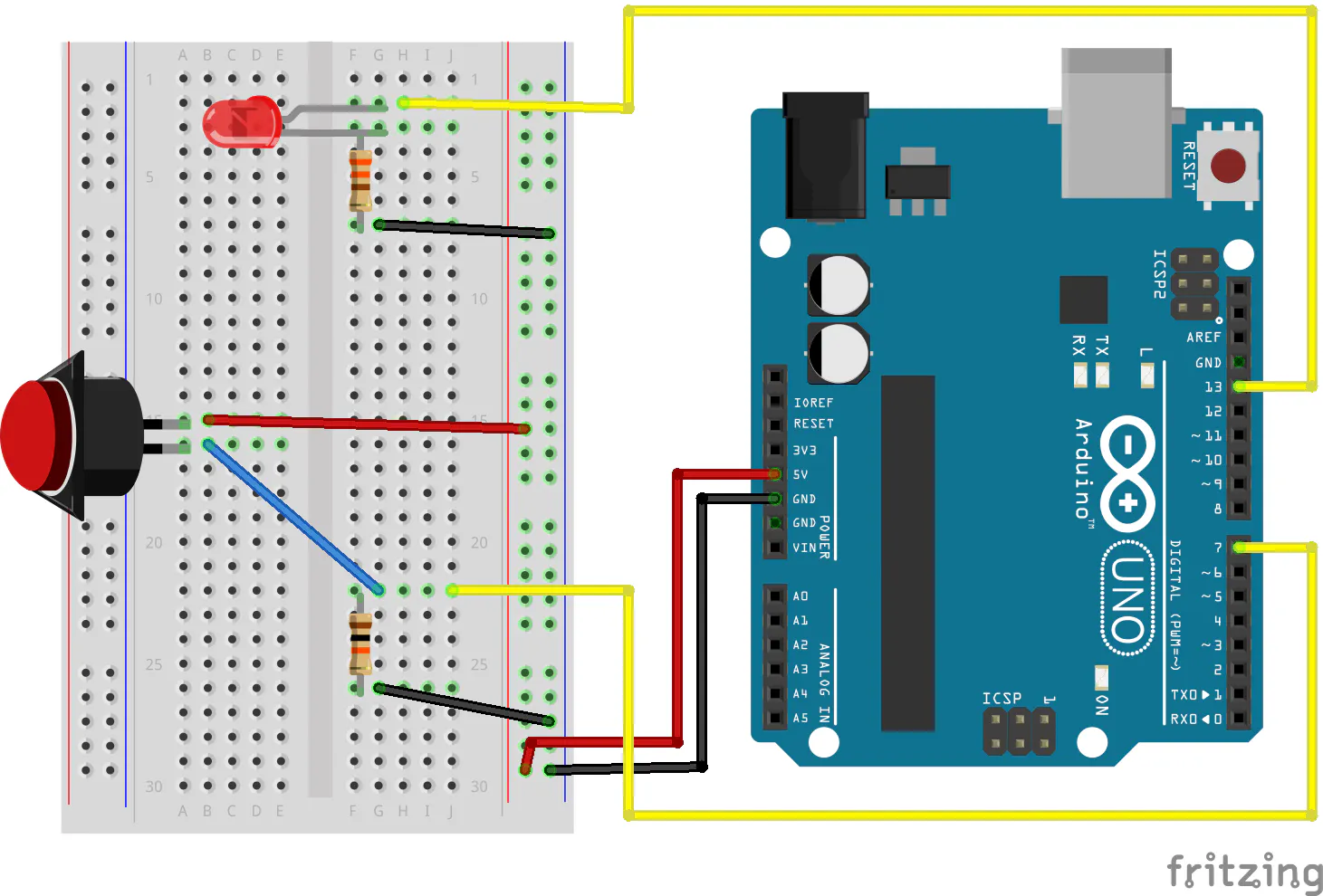Created September 27, 2015

# Homework 3: Automata (and Arduino Examples)

Pressing button, cutting wood, and stressing out.

BeginnerShowcase (no instructions)485## Schematics

### Circuit 1## Code

### Example 1

C/C++
```const int LED = 13;
const int BUTTON = 7;
int val = 0;

void setup() {
pinMode(LED, OUTPUT);
pinMode(BUTTON, INPUT);
}

void loop(){
if (val == HIGH) {
digitalWrite(LED, HIGH);
} else {
digitalWrite(LED, LOW);
}
}
```

### Example 2

C/C++
```const int LED = 13;
const int BUTTON = 7;
int val = 0;
int state = 0;

void setup() {
pinMode(LED, OUTPUT);
pinMode(BUTTON, INPUT);
}

void loop() {
if (val == HIGH) {
state = 1 - state;
}

if (state == 1) {
digitalWrite(LED, HIGH);
} else {
digitalWrite(LED, LOW);
}
}
```

### Example 3

C/C++
```const int LED = 13;
const int BUTTON = 7;
int val = 0;
int old_val = 0;
int state = 0;

void setup() {
pinMode(LED, OUTPUT);
pinMode(BUTTON, INPUT);
}
void loop(){
if ((val == HIGH) && (old_val == LOW)){
state = 1 - state;
}

old_val = val;

if (state == 1) {
digitalWrite(LED, HIGH);
} else {
digitalWrite(LED, LOW);
}
}
```

## Credits

### Eric Chen

9 projects • 1 follower
EECS student at UC Berkeley